Home Practice
For learners and parents For teachers and schools
Textbooks
Full catalogue
Pricing SupportLog in

We think you are located in United States. Is this correct?

# 2.3 Newton's laws

## 2.3 Newton's laws (ESBKR)

In this section we will look at the effect of forces on objects and how we can make things move. This will link together what you have learnt about motion and what you have learnt about forces.

### Newton's first law (ESBKS)

Sir Isaac Newton was a scientist who lived in England (1642-1727) who was interested in the motion of objects under various conditions. He suggested that a stationary object will remain stationary unless a force acts on it and that a moving object will continue moving unless a force slows it down, speeds it up or changes its direction of motion. From this he formulated what is known as Newton's first law of motion:

Newton's first law of motion

An object continues in a state of rest or uniform motion (motion with a constant velocity) unless it is acted on by an unbalanced (net or resultant) force.

This property of an object, to continue in its current state of motion unless acted upon by a net force, is called inertia.

Let us consider the following situations:

An ice skater pushes herself away from the side of the ice rink and skates across the ice. She will continue to move in a straight line across the ice unless something stops her. Objects are also like that. If we kick a soccer ball across a soccer field, according to Newton's first law, the soccer ball should keep on moving forever! However, in real life this does not happen. Is Newton's Law wrong? Not really. Newton's first law applies to situations where there aren't any external forces present. This means that friction is not present. In the case of the ice skater, the friction between the skates and the ice is very little and she will continue moving for quite a distance. In the case of the soccer ball, air resistance (friction between the air and the ball) and friction between the grass and the ball is present and this will slow the ball down.

#### Newton's first law in action

Let us look at the following two examples. We will start with a familiar example:

Seat belts:

We wear seat belts in cars. This is to protect us when the car is involved in an accident. If a car is travelling at $$\text{120}$$ $$\text{km·h^{-1}}$$, the passengers in the car is also travelling at $$\text{120}$$ $$\text{km·h^{-1}}$$. When the car suddenly stops a force is exerted on the car (making it slow down), but not on the passengers. The passengers will carry on moving forward at $$\text{120}$$ $$\text{km·h^{-1}}$$ according to Newton's first law. If they are wearing seat belts, the seat belts will stop them by exerting a force on them and so prevent them from getting hurt.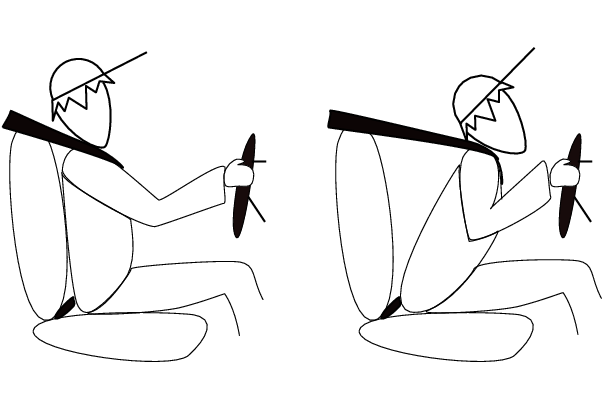Rockets:

A spaceship is launched into space. The force of the exploding gases pushes the rocket through the air into space. Once it is in space, the engines are switched off and it will keep on moving at a constant velocity. If the astronauts want to change the direction of the spaceship they need to fire an engine. This will then apply a force on the rocket and it will change its direction.

## Worked example 8: Newton's first law in action

Why do passengers get thrown to the side when the car they are driving in goes around a corner?

### What happens before the car turns

Before the car starts turning both the passengers and the car are travelling at the same velocity. (picture A)

### What happens while the car turns

The driver turns the wheels of the car, which then exert a force on the car and the car turns. This force acts on the car but not the passengers, hence (by Newton's first law) the passengers continue moving with the same original velocity. (picture B)

### Why passengers get thrown to the side?

If the passengers are wearing seat belts they will exert a force on the passengers until the passengers' velocity is the same as that of the car (picture C). Without a seat belt the passenger may hit the side of the car.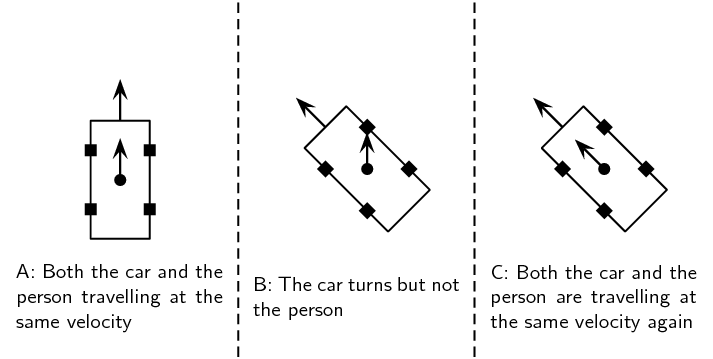Textbook Exercise 2.4

If a passenger is sitting in a car and the car turns round a bend to the right, what happens to the passenger? What happens if the car turns to the left?

Before the car starts turning both the passenger and the car are travelling at the same velocity.

As the car turns to the right a force acts on the car but not the passengers, hence (by Newton's first law) the passenger continues moving with the same original velocity. (In other words the car turns, but the passenger does not).

The net result of this is that the passenger is pulled to the left as the car turns right.

If the car instead turned to the left the passenger would be pulled to the right.

Helium is less dense than the air we breathe. Discuss why a helium balloon in a car driving around a corner appears to violate Newton's first law and moves towards the inside of the turn not the outside like a passenger.

As the car goes around the corner all the air keeps moving forward (it acts in the same way that a passenger acts). This causes the air pressure on the one side of the car to increase (this will be on the opposite side to the direction the car is turning). This slight increase in the air pressure pushes the helium balloon to the other side of the car.

Because of this it appears that the helium balloon does not obey Newton's first law.

### Newton's second law of motion (ESBKT)

According to Newton's first law, things 'like to keep on doing what they are doing'. In other words, if an object is moving, it tends to continue moving (in a straight line and at the same speed) and if an object is stationary, it tends to remain stationary. So how do objects start moving?

Let us look at the example of a $$\text{10}$$ $$\text{kg}$$ box on a rough table. If we push lightly on the box as indicated in the diagram, the box won't move. Let's say we applied a force of $$\text{100}$$ $$\text{N}$$, yet the box remains stationary. At this point a frictional force of $$\text{100}$$ $$\text{N}$$ is acting on the box, preventing the box from moving. If we increase the force, let's say to $$\text{150}$$ $$\text{N}$$ and the box almost starts to move, the frictional force is $$\text{150}$$ $$\text{N}$$. To be able to move the box, we need to push hard enough to overcome the friction and then move the box. If we therefore apply a force of $$\text{200}$$ $$\text{N}$$ remembering that a frictional force of $$\text{150}$$ $$\text{N}$$ is present, the 'first' $$\text{150}$$ $$\text{N}$$ will be used to overcome or 'cancel' the friction and the other $$\text{50}$$ $$\text{N}$$ will be used to move (accelerate) the block. In order to accelerate an object we must have a resultant force acting on the block.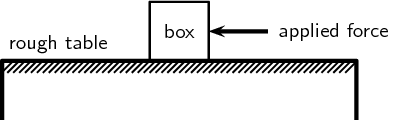Now, what do you think will happen if we pushed harder, lets say $$\text{300}$$ $$\text{N}$$? Or, what do you think will happen if the mass of the block was more, say $$\text{20}$$ $$\text{kg}$$, or what if it was less? Let us investigate how the motion of an object is affected by mass and force.

A recommended experiment for formal assessment on Newton's second law of motion is also included in this chapter. In this experiment learners will investigate the relationship between force and acceleration (Newton's second law). You will need trolleys, different masses, inclined plane, rubber bands, meter ruler, ticker tape apparatus, ticker timer, graph paper.

## Newton's second law of motion

### Aim

To investigate the relation between the acceleration of objects and the application of a constant resultant force.### Method

1. A constant force of $$\text{20}$$ $$\text{N}$$, acting at an angle of $$\text{60}$$$$\text{°}$$ to the horizontal, is applied to a dynamics trolley.

2. Ticker tape attached to the trolley runs through a ticker timer of frequency $$\text{20}$$ $$\text{Hz}$$ as the trolley is moving on the frictionless surface.

3. The above procedure is repeated 4 times, each time using the same force, but varying the mass of the trolley as follows:

• Case 1: $$\text{6,25}$$ $$\text{kg}$$

• Case 2: $$\text{3,57}$$ $$\text{kg}$$

• Case 3: $$\text{2,27}$$ $$\text{kg}$$

• Case 4: $$\text{1,67}$$ $$\text{kg}$$

4. Shown below are sections of the four ticker tapes obtained. The tapes are marked with the letters A, B, C, D, etc. A is the first dot, B is the second dot and so on. The distance between each dot is also shown.

Instructions:

1. Use each tape to calculate the instantaneous velocity (in $$\text{m·s^{-1}}$$) of the trolley at points B and F (remember to convert the distances to m first!). Use these velocities to calculate the trolley's acceleration in each case.

2. Tabulate the mass and corresponding acceleration values as calculated in each case. Ensure that each column and row in your table is appropriately labelled.

3. Draw a graph of acceleration vs. mass, using a scale of $$\text{1}$$ $$\text{cm}$$ = $$\text{1}$$ $$\text{m·s^{-2}}$$ on the y-axis and $$\text{1}$$ $$\text{cm}$$ = $$\text{1}$$ $$\text{kg}$$ on the x-axis.

4. Use your graph to read off the acceleration of the trolley if its mass is $$\text{5}$$ $$\text{kg}$$.

5. Write down a conclusion for the experiment.

You will have noted in the investigation above that the heavier the trolley is, the slower it moved when the force was constant. The acceleration is inversely proportional to the mass. In mathematical terms:

$$a\propto \frac{1}{m}$$

In a similar investigation where the mass is kept constant, but the applied force is varied, you will find that the bigger the force is, the faster the object will move. The acceleration of the trolley is therefore directly proportional to the resultant force. In mathematical terms:

$$a\propto F.$$

Rearranging the above equations, we get a $$\propto$$$$\frac{F}{m}$$ or $$F = ma$$.

Remember that both force and acceleration are vectors quantities. The acceleration is in the same direction as the force that is being applied. If multiple forces are acting simultaneously then we only need to work with the resultant force or net force.

Newton's second law of motion

If a resultant force acts on a body, it will cause the body to accelerate in the direction of the resultant force. The acceleration of the body will be directly proportional to the resultant force and inversely proportional to the mass of the body. The mathematical representation is:$\vec{F}_{net} = m\vec{a}$

Force is a vector quantity. Newton's second law of motion should be applied to the $$y$$- and $$x$$-directions separately. You can use the resulting $$y$$- and $$x$$-direction resultants to calculate the overall resultant as we saw in the previous chapter.

temp text

#### Applying Newton's second law of motion

Newton's second law can be applied to a variety of situations. We will look at the main types of examples that you need to study.

## Worked example 9: Newton's second law: Box on a surface

A $$\text{10}$$ $$\text{kg}$$ box is placed on a table. A horizontal force of magnitude $$\text{32}$$ $$\text{N}$$ is applied to the box. A frictional force of magnitude $$\text{7}$$ $$\text{N}$$ is present between the surface and the box.

1. Draw a force diagram indicating all of the forces acting on the box.

2. Calculate the acceleration of the box.

### Identify the horizontal forces and draw a force diagram

We only look at the forces acting in a horizontal direction (left-right) and not vertical (up-down) forces. The applied force and the force of friction will be included. The force of gravity, which is a vertical force, will not be included.

### Calculate the acceleration of the box

Remember that we consider the $$y$$- and $$x$$-directions separately. In this problem we can ignore the $$y$$-direction because the box is resting on a table with the gravitational force balanced by the normal force.

We have been given:

Applied force $${F}_{1}=\text{32}\text{ N}$$

Frictional force $${F}_{f}=-\text{7}\text{ N}$$

Mass $$m=\text{10}\text{ kg}$$

To calculate the acceleration of the box we will be using the equation $$\vec{F}_{R}=m\vec{a}$$. Therefore: \begin{align*} \vec{F}_R &= m\vec{a} \\ \vec{F}_1+\vec{F}_f &= (\text{10})\vec{a} \\ (\text{32}-\text{7}) &= (\text{10})\vec{a} \\ \text{25} &= (\text{10})\vec{a} \\ \vec{a} &= \text{2,5}\text{ m·s$^{-2}$}\ \text{to the left.} \end{align*}

## Worked example 10: Newton's second law: box on a surface

Two crates, $$\text{10}$$ $$\text{kg}$$ and $$\text{15}$$ $$\text{kg}$$ respectively, are connected with a thick rope according to the diagram. A force, to the right, of $$\text{500}$$ $$\text{N}$$ is applied. The boxes move with an acceleration of $$\text{2}$$ $$\text{m·s^{-2}}$$ to the right. One third of the total frictional force is acting on the $$\text{10}$$ $$\text{kg}$$ block and two thirds on the $$\text{15}$$ $$\text{kg}$$ block. Calculate:

1. the magnitude and direction of the total frictional force present.

2. the magnitude of the tension in the rope at T.

Important: when you have tension in a rope in a problem like this you need to know that both ends of the rope apply a force with the same magnitude but opposite direction. We call this force the tension and you should examine the force diagrams in this problem carefully.

### Evaluate what is given

To make things easier lets give the two crates labels, let us call the $$\text{10}$$ $$\text{kg}$$ crate number 2 and the $$\text{15}$$ $$\text{kg}$$ crate number 1.

We have two crates that have overall has an acceleration that is given. The fact that the crates are tied together with a rope means that they will both have the same acceleration. They will also both feel the same force due to the tension in the rope.

We are told that there is friction but we are only given the relationship between the total frictional force both crates experience and the fraction each one experiences. The total friction, $$\vec{F}_{fT}$$ will be the sum of the friction on crate 1, $$\vec{F}_{f1}$$, and the friction on crate 2, $$\vec{F}_{f2}$$. We are told that $$\vec{F}_{f1}=\frac{\text{2}}{\text{3}}\vec{F}_{fT}$$ and $$\vec{F}_{f2}=\frac{\text{1}}{\text{3}}\vec{F}_{fT}$$. We know the blocks are accelerating to the right and we know that friction will be in the direction opposite to the direction of motion and parallel to the surface.

### Draw force diagrams

The diagram for crate 1 will be:The diagram for crate 1 (indicated by blue dotted lines) will be:Where:

• $$\vec{F}_{g1}$$ is the force due to gravity on the first crate
• $$\vec{N}_{1}$$ is the normal force from the surface on the first crate
• $$\vec{T}$$ is the force of tension in the rope
• $$\vec{F}_{applied}$$ is the external force being applied to the crate
• $$\vec{F}_{f1}$$ is the force of friction on the first crate

The diagram for crate 2 (indicated by orange dashed lines) will be: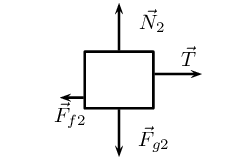Where:

• $$\vec{F}_{g2}$$ is the force due to gravity on the second crate
• $$\vec{N}_{2}$$ is the normal force from the surface on the second crate
• $$\vec{T}$$ is the force of tension in the rope
• $$\vec{F}_{f2}$$ is the force of friction on the second crate

### Apply Newton's second law of motion

The problem tells us that the crates are accelerating along the $$x$$-direction which means that the forces in the $$y$$-direction do not result in a net force. We can treat the different directions separately so we only need to consider the $$x$$-direction.

We are working with one dimension and can choose a sign convention to indicate the direction of the vectors. We choose vectors to the right (or in the positive $$x$$-direction) to be positive.

We can now apply Newton's second law of motion to the first crate because we know the acceleration and we know all the forces acting on the crate. Using positive to indicate a force to the right we know that $${F}_{res1} = F_{applied}-{F}_{f1}-T$$ \begin{align*} \vec{F}_{res1} &=m_1\vec{a} \\ F_{applied}-{F}_{f1}-T &=m_1a \\ F_{applied}-\frac{\text{2}}{\text{3}}{F}_{fT}-T &=m_1a \\ (500)-\frac{\text{2}}{\text{3}}{F}_{fT}-T &=(\text{15})(2) \\ -T &=(\text{15})(2) -(500)+\frac{\text{2}}{\text{3}}{F}_{fT} \end{align*}

Now apply Newton's second law of motion to the second crate because we know the acceleration and we know all the forces acting on the crate. We know that $${F}_{res2} = T-{F}_{f2}$$. Note that tension is in the opposite direction. \begin{align*} \vec{F}_{res2} &=m_2\vec{a} \\ T-{F}_{f2} &=m_2a \\ T - \frac{\text{1}}{\text{3}}{F}_{fT} &=m_2a \\ T &=(\text{10})(2) +\frac{\text{1}}{\text{3}}{F}_{fT} \end{align*}

### Solve simultaneously

We have used Newton's second law of motion to create two equations with two unknowns, this means we can solve simultaneously. We solved for $$T$$ in the equations above but one carries a negative sign so if we add the two equations we will subtract out the value of the tension allowing us to solve for $${F}_{fT}$$: \begin{align*} (T) + (-T) &= ((\text{10})(2) +\frac{\text{1}}{\text{3}}{F}_{fT}) + ((\text{15})(2) -(500)+\frac{\text{2}}{\text{3}}{F}_{fT}) \\ 0 & = \text{20} + \text{30} - \text{500} + \frac{\text{1}}{\text{3}}{F}_{fT} + \frac{\text{2}}{\text{3}}{F}_{fT} \\ 0 & = -\text{450} + {F}_{fT} \\ {F}_{fT} & = \text{450}\text{ N} \end{align*}

We can substitute the magnitude of $${F}_{fT}$$ into the equation for crate 2 to determine the magnitude of the tension: \begin{align*} T &=(\text{10})(2) +\frac{\text{1}}{\text{3}}{F}_{fT} \\ T &=(\text{10})(2) +\frac{\text{1}}{\text{3}}(\text{450}) \\ T &= \text{20} +\text{150} \\ T &= \text{170}\text{ N} \end{align*}

The total force due to friction is $$\text{450}$$ $$\text{N}$$ to the left. The magnitude of the force of tension is $$\text{170}$$ $$\text{N}$$.

## Worked example 11: Newton's second law: box on a surface (Alternative Method)

Two crates, $$\text{10}$$ $$\text{kg}$$ and $$\text{15}$$ $$\text{kg}$$ respectively, are connected with a thick rope according to the diagram. A force, to the right, of $$\text{500}$$ $$\text{N}$$ is applied. The boxes move with an acceleration of $$\text{2}$$ $$\text{m·s^{-2}}$$ to the right. One third of the total frictional force is acting on the $$\text{10}$$ $$\text{kg}$$ block and two thirds on the $$\text{15}$$ $$\text{kg}$$ block. Calculate:

1. the magnitude and direction of the total frictional force present.

2. the magnitude of the tension in the rope at T.

Important: when you have tension in a rope in a problem like this you need to know that both ends of the rope apply a force with the same magnitude but opposite direction. We call this force the tension and you should examine the force diagrams in this problem carefully.

### Draw a force diagram

Always draw a force diagram although the question might not ask for it. The acceleration of the whole system is given, therefore a force diagram of the whole system will be drawn. Because the two crates are seen as a unit, the force diagram will look like this:

### Calculate the frictional force

To find the frictional force we will apply Newton's second law. We are given the mass ($$\text{10}$$ + $$\text{15}$$ $$\text{kg}$$) and the acceleration ($$\text{2}$$ $$\text{m·s^{-2}}$$). Choose the direction of motion to be the positive direction (to the right is positive).

\begin{align*} {F}_{R} & = ma\\ {F}_{\text{applied}}+{F}_{f} & =ma\\ \text{500}+{F}_{f} & =\left(10+15\right)\left(2\right)\\ {F}_{f} & =50-\text{500}\\ {F}_{f} & =-\text{450}N \end{align*}

The frictional force is $$\text{450}$$ $$\text{N}$$ opposite to the direction of motion (to the left).

### Find the tension in the rope

To find the tension in the rope we need to look at one of the two crates on their own. Let's choose the $$\text{10}$$ $$\text{kg}$$ crate. Firstly, we need to draw a force diagram: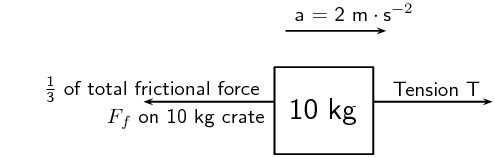Figure 2.5: Force diagram of $$\text{10}$$ $$\text{kg}$$ crate.

The frictional force on the $$\text{10}$$ $$\text{kg}$$ block is one third of the total, therefore:

$${F}_{f}=\frac{\text{1}}{\text{3}}\times \text{450}$$

$${F}_{f}=\text{150} \text{N}$$

If we apply Newton's second law:

\begin{align*} {F}_{R}& = ma \\ T+{F}_{f}& = \left(10\right)\left(2\right) \\ T+\left(-\text{150}\right)& = 20 \\ T& = \text{170}\text{ N} \end{align*}

Note: If we had used the same principle and applied it to $$\text{15}$$ $$\text{kg}$$ crate, our calculations would have been the following:

\begin{align*} {F}_{R}& = ma \\ {F}_{\text{applied}}+T+{F}_{f}& = \left(15\right)\left(2\right) \\ \text{500}+T+\left(-\text{300}\right)& = 30 \\ T& = -\text{170}\text{ N} \end{align*}

The negative answer here means that the force is in the direction opposite to the motion, in other words to the left, which is correct. However, the question asks for the magnitude of the force and your answer will be quoted as $$\text{170}$$ $$\text{N}$$.

## Worked example 12: Newton's second law: man pulling a box

A man is pulling a $$\text{20}$$ $$\text{kg}$$ box with a rope that makes an angle of $$\text{60}$$$$\text{°}$$ with the horizontal. If he applies a force of magnitude $$\text{150}$$ $$\text{N}$$ and a frictional force of magnitude $$\text{15}$$ $$\text{N}$$ is present, calculate the acceleration of the box.

### Draw a force diagram or free body diagram

The motion is horizontal and therefore we will only consider the forces in a horizontal direction. Remember that vertical forces do not influence horizontal motion and vice versa.

### Calculate the horizontal component of the applied force

We first need to choose a direction to be the positive direction in this problem. We choose the positive $$x$$-direction (to the right) to be positive.

The applied force is acting at an angle of $$\text{60}$$$$\text{°}$$ to the horizontal. We can only consider forces that are parallel to the motion. The horizontal component of the applied force needs to be calculated before we can continue: \begin{align*} F_x & = F_{applied}\cos(\theta) \\ &= \text{150}\cos(\text{60}\text{°}) \\ & = \text{75}\text{ N} \end{align*}

### Calculate the acceleration

To find the acceleration we apply Newton's second law: \begin{align*} F_R&=ma \\ F_x+F_f&=(\text{20})a \\ (\text{75}) + (-\text{15})&= (\text{20})a\\ a & = \frac{\text{60}}{\text{20}} \\ a & = \text{3}\text{ m·s$^{-2}$} \end{align*}The acceleration is $$\text{3}$$ $$\text{m·s^{-2}}$$ to the right.

## Worked example 13: Newton's second law: truck and trailer

A $$\text{2 000}$$ $$\text{kg}$$ truck pulls a $$\text{500}$$ $$\text{kg}$$ trailer with a constant acceleration. The engine of the truck produces a thrust of $$\text{10 000}$$ $$\text{N}$$. Ignore the effect of friction. Calculate the:

1. acceleration of the truck; and

2. tension in the tow bar T between the truck and the trailer, if the tow bar makes an angle of $$\text{25}$$$$\text{°}$$ with the horizontal.

### Draw a force diagram

Draw a force diagram indicating all the forces on the system as a whole:

### Apply Newton's second law of motion

We choose the positive $$x$$-direction to be the positive direction. We only need to consider the horizontal forces. Using only the horizontal forces means that we first need to note that the tension acts at an angle to the horizontal and we need to use the horizontal component of the tension in our calculations.The horizontal component has a magnitude of $$T\cos(\text{25}\text{°})$$.

In the absence of friction, the only force that causes the system to accelerate is the thrust of the engine. If we now apply Newton's second law of motion to the truck we have: \begin{align*} \vec{F}_{Rtruck} &= m_{truck}\vec{a}\ \text{(we use signs to indicate direction)} \\ {F}_{engine} - T\cos(\text{25}\text{°}) &= (\text{2 000})a \\ (\text{10 000}) - T\cos(\text{25}\text{°}) &= (\text{2 000})a \\ a & = \frac{(\text{10 000}) - T\cos(\text{25}\text{°})}{(\text{2 000})} \end{align*}

We now apply the same principle to the trailer (remember that the direction of the tension will be opposite to the case of the truck): \begin{align*} \vec{F}_{Rtrailer} &= m_{trailer}\vec{a}\ \text{(we use signs to indicate direction)} \\ T\cos(\text{25}\text{°}) &= (\text{500})a \\ a & = \frac{T\cos(\text{25}\text{°})}{(\text{500})} \end{align*}

We now have two equations and two unknowns so we can solve simultaneously. We subtract the second equation from the first to get: \begin{align*} (a) - (a) &=(\frac{(\text{10 000}) - T\cos(\text{25}\text{°})}{(\text{2 000})}) - (\frac{T\cos(\text{25}\text{°})}{(\text{500})}) \\ 0 & = (\frac{(\text{10 000}) - T\cos(\text{25}\text{°})}{(\text{2 000})}) - (\frac{T\cos(\text{25}\text{°})}{(\text{500})})\\ & \text{(multiply through by \text{2 000})} \\ 0 & = (\text{10 000}) - T\cos(\text{25}\text{°}) - 4T\cos(\text{25}\text{°}) \\ \text{5}T\cos(\text{25}\text{°}) & = (\text{10 000}) \\ T & = \frac{(\text{10 000})}{\text{5}\cos(\text{25}\text{°})} \\ T & = \text{2 206,76}\text{ N} \end{align*}

Now substitute this result back into the second equation to solve for the magnitude of $$a$$ \begin{align*} a & = \frac{T\cos(\text{25}\text{°})}{(\text{500})}\\ & = \frac{(\text{2 206,76})\cos(\text{25}\text{°})}{(\text{500})}\\ & = \text{4,00}\text{ m·s$^{-2}$} \end{align*}

temp text

#### Object on an inclined plane

In an earlier section we looked at the components of the gravitational force parallel and perpendicular to the slope for objects on an inclined plane. When we look at problems on an inclined plane we need to include the component of the gravitational force parallel to the slope.

Think back to the pictures of the book on a table, as one side of the table is lifted higher the book starts to slide. Why? The book starts to slide because the component of the gravitational force parallel to the surface of the table gets larger for the larger angle of inclination. This is like the applied force and it eventually becomes larger than the frictional force and the book accelerates down the table or inclined plane.

The force of gravity will also tend to push an object 'into' the slope. This is the component of the force perpendicular to the slope. There is no movement in this direction as this force is balanced by the slope pushing up against the object. This “pushing force” is the normal force (N) which we have already learnt about and is equal in magnitude to the perpendicular component of the gravitational force, but opposite in direction.

Do not use the abbreviation $$W$$ for weight as it is used to abbreviate 'work'. Rather use the force of gravity $${F}_{g}$$ for weight.

## Worked example 14: Newton's second law: box on inclined plane

A body of mass $$M$$ is at rest on an inclined plane due to friction.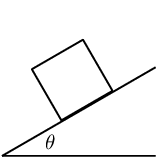What of the following option is the magnitude of the frictional force acting on the body?

1. $$F_g$$

2. $$F_g\cos(θ)$$

3. $$F_g\sin(θ)$$

4. $$F_g\tan(θ)$$

### Analyse the situation

The question asks us to determine the magnitude of the frictional force. The body is said to be at rest on the plane, which means that it is not moving and therefore the acceleration is zero. We know that the frictional force will act parallel to the slope. If there were no friction the box would slide down the slope so friction must be acting up the slope. We also know that there will be a component of gravity perpendicular to the slope and parallel to the slope. The free body diagram for the forces acting on the block is: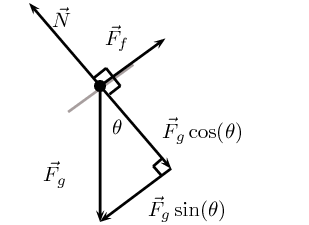### Determine the magnitude of the frictional force

We can apply Newton's second law to this problem. We know that the object is not moving so the resultant acceleration is zero. We choose up the slope to be the positive direction. Therefore: \begin{align*} \vec{F}_R &= m\vec{a}\; \text{using signs for direction}\\ F_f - F_g\sin(\theta) &= m (0)\\ F_f - F_g\sin(\theta) &= m (0)\\ F_f & = F_g\sin(\theta) \end{align*}

The force of friction has the same magnitude as the component of the force of gravitation parallel to the slope, $$F_g\sin(\theta)$$.

## Worked example 15: Newton's second law: object on an incline

A force of magnitude $$T=\text{312} \text{N}$$ up an incline is required to keep a body at rest on a frictionless inclined plane which makes an angle of $$\text{35}$$$$\text{°}$$ with the horizontal. Calculate the magnitudes of the force due to gravity and the normal force, giving your answers to three significant figures.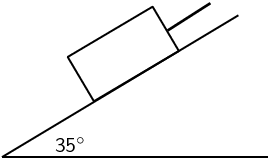### Find the magnitude of $$\vec{F}_g$$

We are usually asked to find the magnitude of $$\vec{T}$$, but in this case $$\vec{T}$$ is given and we are asked to find $$\vec{F}_g$$. We can use the same equation. $$T$$ is the force that balances the component of $$\vec{F}_g$$ parallel to the plane ($${F}_{gx}$$) and therefore it has the same magnitude.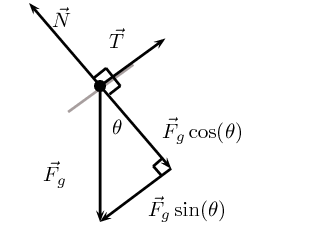We can apply Newton's second law to this problem. We know that the object is not moving so the resultant acceleration is zero. We choose up the slope to be the positive direction. Therefore: \begin{align*} \vec{F}_R &= m\vec{a}\; \text{using signs for direction}\\ T - F_g\sin(\theta) &= m (0)\\ F_g & = \frac{T}{\sin(\theta)} \\ & = \frac{\text{312}}{\sin(\text{35}\text{°})} \\ & = \text{543,955}\text{ N} \end{align*}

### Find the magnitude of $$\vec{N}$$

We treat the forces parallel and perpendicular to the slope separately. The block is stationary so the acceleration perpendicular to the slope is zero. Once again we can apply Newton's second law of motion. We choose the direction of the normal force as the positive direction. \begin{align*} \vec{F}_R &= m\vec{a}\; \text{using signs for direction}\\ N - F_g\cos(\theta) &= m (0)\\ N & = F_g\cos(\theta) \end{align*}

We could substitute in the value of $$F_g$$ calculated earlier. We would like to illustrate that there is another approach to adopt to ensure you get the correct answer even if you made a mistake calculating $$F_g$$. $$F_g\cos(\theta)$$ can also be determined with the use of trigonometric ratios. We know from the previous part of the question that $$T = F_g\sin(\theta)$$. We also know that \begin{align*} \tan(\theta) &=\frac{F_g\sin(\theta)}{F_g\cos(\theta)}\\ &=\frac{T}{N}\\ N&=\frac{T}{\tan(\theta)}\\ & = \frac{\text{312}}{\tan(\text{35}\text{°})} \\ & = \text{445,58}\text{ N} \end{align*}

Note that the question asks that the answers be given to 3 significant figures. We therefore round $$\vec{N}$$ from $$\text{445,58}$$ $$\text{N}$$ up to $$\text{446}$$ $$\text{N}$$ perpendicular to the surface upwards and $$\vec{T}$$ from $$\text{543,955}$$ $$\text{N}$$ up to $$\text{544}$$ $$\text{N}$$ parallel to the plane, up the slope.

temp text

#### Lifts and rockets

So far we have looked at objects being pulled or pushed across a surface, in other words motion parallel to the surface the object rests on. Here we only considered forces parallel to the surface, but we can also lift objects up or let them fall. This is vertical motion where only vertical forces are being considered.

Let us consider a $$\text{500}$$ $$\text{kg}$$ lift, with no passengers, hanging on a cable. The purpose of the cable is to pull the lift upwards so that it can reach the next floor or lower the lift so that it can move downwards to the floor below. We will look at five possible stages during the motion of the lift and apply our knowledge of Newton's second law of motion to the situation. The 5 stages are:

1. A stationary lift suspended above the ground.
2. A lift accelerating upwards.
3. A lift moving at a constant velocity.
4. A lift decelerating (slowing down).
5. A lift accelerating downwards (the cable snaps!).

We choose the upwards direction to be the positive direction for this discussion.

Stage 1:

The $$\text{500}$$ $$\text{kg}$$ lift is stationary at the second floor of a tall building.

The lift is not accelerating. There must be a tension $$\vec{T}$$ from the cable acting on the lift and there must be a force due to gravity, $$\vec{F}_g$$. There are no other forces present and we can draw the free body diagram: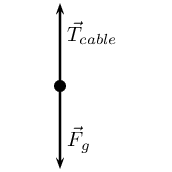We apply Newton's second law to the vertical direction: \begin{align*} \vec{F}_R & = m_{\text{lift}} \vec{a}\ \text{(we use signs to indicate direction)} \\ T - F_g & = m_{\text{lift}} (0) \\ T & = F_g \end{align*}

The forces are equal in magnitude and opposite in direction.

Stage 2:

The lift moves upwards at an acceleration of $$\text{1}$$ $$\text{m·s^{-2}}$$.

If the lift is accelerating, it means that there is a resultant force in the direction of the motion. This means that the force acting upwards is now greater than the force due to gravity $$\vec{F}_g$$ (down). To find the magnitude of the $$\vec{T}$$ applied by the cable we can do the following calculation: (Remember we have chosen upwards as positive.)

We apply Newton's second law to the vertical direction: \begin{align*} \vec{F}_R & = m_{\text{lift}} \vec{a}\ \text{(we use signs to indicate direction)} \\ T - F_g & = m_{\text{lift}} (\text{1}) \\ T & = F_g + m_{\text{lift}} (\text{1}) \end{align*}

The answer makes sense as we need a bigger force upwards to cancel the effect of gravity as well as have a positive resultant force.

Stage 3:

The lift moves at a constant velocity.

When the lift moves at a constant velocity, the acceleration is zero, \begin{align*} \vec{F}_R & = m_{\text{lift}} \vec{a}\ \text{(we use signs to indicate direction)} \\ T - F_g & = m_{\text{lift}} (0) \\ T & = F_g \end{align*}

The forces are equal in magnitude and opposite in direction. It is common mistake to think that because the lift is moving there is a net force acting on it. It is only if it is accelerating that there is a net force acting.

Stage 4:

The lift slows down at a rate of $$\text{2}$$ $$\text{m·s^{-2}}$$. The lift was moving upwards so this means that it is decelerating or accelerating in the direction opposite to the direction of motion. This means that the acceleration is in the negative direction. \begin{align*} \vec{F}_R & = m_{\text{lift}} \vec{a}\ \text{(we use signs to indicate direction)} \\ T - F_g & = m_{\text{lift}} (-\text{2}) \\ T & = F_g -\text{2}m_{\text{lift}} \end{align*}

As the lift is now slowing down there is a resultant force downwards. This means that the force acting downwards is greater than the force acting upwards.

This makes sense as we need a smaller force upwards to ensure that the resultant force is downward. The force of gravity is now greater than the upward pull of the cable and the lift will slow down.

Stage 5:

The cable snaps.

When the cable snaps, the force that used to be acting upwards is no longer present. The only force that is present would be the force of gravity. The lift will fall freely and its acceleration.

#### Apparent weight

Your weight is the magnitude of the gravitational force acting on your body. When you stand in a lift that is stationery and then starts to accelerate upwards you feel you are pressed into the floor while the lift accelerates. You feel like you are heavier and your weight is more. When you are in a stationery lift that starts to accelerate downwards you feel lighter on your feet. You feel like your weight is less.

Weight is measured through normal forces. When the lift accelerates upwards you feel a greater normal force acting on you as the force required to accelerate you upwards in addition to balancing out the gravitational force.

When the lift accelerates downwards you feel a smaller normal force acting on you. This is because a net force downwards is required to accelerate you downwards. This phenomenon is called apparent weight because your weight didn't actually change.

temp text

#### Rockets

As with lifts, rockets are also examples of objects in vertical motion. The force of gravity pulls the rocket down while the thrust of the engine pushes the rocket upwards. The force that the engine exerts must overcome the force of gravity so that the rocket can accelerate upwards. The worked example below looks at the application of Newton's second law in launching a rocket.

## Worked example 16: Newton's second law: rocket

A rocket (of mass $$\text{5 000}$$ $$\text{kg}$$) is launched vertically upwards into the sky at an acceleration of $$\text{20}$$ $$\text{m·s^{-2}}$$. If the magnitude of the force due to gravity on the rocket is $$\text{49 000}$$ $$\text{N}$$, calculate the magnitude and direction of the thrust of the rocket's engines.

### Analyse what is given and what is asked

We have the following:

$$m=\text{5 000} \text{kg}$$

$$\vec{a}$$=$$\text{20}$$ $$\text{m·s^{-2}}$$ upwards.

$$\vec{F}_g$$=$$\text{49 000}$$ $$\text{N}$$ downwards.

We are asked to find the thrust of the rocket engine $$\vec{F}$$.

### Find the thrust of the engine

We will apply Newton's second law: \begin{align*} \vec{F}_R & = m\vec{a}\ \text{(using signs to indicate direction)} \\ F - F_g & = (\text{5 000})(\text{20}) \\ F - (\text{49 000}) & = (\text{5 000})(\text{20}) \\ F &= \text{149 000}\text{ N} \end{align*}

The force due to the thrust is $$\text{149 000}$$ $$\text{N}$$ upwards.

## Worked example 17: Rockets

How do rockets accelerate in space?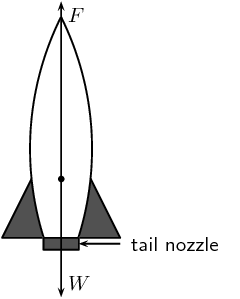### Gas explodes inside the rocket. This exploding gas exerts a force on each side of the rocket (as shown in the picture below of the explosion chamber inside the rocket).Due to the symmetry of the situation, all the forces exerted on the rocket are balanced by forces on the opposite side, except for the force opposite the open side. This force on the upper surface is unbalanced. This is therefore the resultant force acting on the rocket and it makes the rocket accelerate forwards.

Textbook Exercise 2.5

A tug is capable of pulling a ship with a force of $$\text{100}$$ $$\text{kN}$$. If two such tugs are pulling on one ship, they can produce any force ranging from a minimum of $$\text{0}$$ $$\text{kN}$$ to a maximum of $$\text{200}$$ $$\text{kN}$$. Give a detailed explanation of how this is possible. Use diagrams to support your result.

We start off with the two tug boats pulling in opposite directions: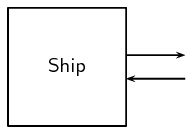The resultant force is $$\text{0}$$ $$\text{kN}$$ since the tug boats are pulling with equal forces in opposite directions.

If the two tugboats pull in the same direction then we get:The resultant force is $$\text{200}$$ $$\text{kN}$$ since the tug boats are pulling with equal forces in the same direction.

To get any force between these two extremes, one tugboat will have to be pulling on the ship at a different angle to the second tugboat, for example: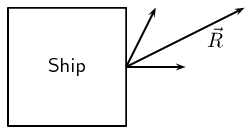Note that the resultant force in this situation is less than $$\text{200}$$ $$\text{kN}$$ (You can check this by using any of the vector addition techniques).

A car of mass $$\text{850}$$ $$\text{kg}$$ accelerates at $$\text{2}$$ $$\text{m·s^{-2}}$$. Calculate the magnitude of the resultant force that is causing the acceleration.

\begin{align*} F & = ma \\ & = (\text{850})(\text{2}) \\ & = \text{1 700}\text{ N} \end{align*}

Find the force needed to accelerate a $$\text{3}$$ $$\text{kg}$$ object at $$\text{4}$$ $$\text{m·s^{-2}}$$.

\begin{align*} F & = ma \\ & = (\text{3})(\text{4}) \\ & = \text{12}\text{ N} \end{align*}

Calculate the acceleration of an object of mass $$\text{1 000}$$ $$\text{kg}$$ accelerated by a force of magnitude $$\text{100}$$ $$\text{N}$$.

\begin{align*} F & = ma \\ a & = \frac{F}{m} \\ & = \frac{\text{100}}{\text{1 000}} \\ & = \text{0,1}\text{ m·s$^{-2}$} \end{align*}

An object of mass $$\text{7}$$ $$\text{kg}$$ is accelerating at $$\text{2,5}$$ $$\text{m·s^{-2}}$$. What resultant force acts on it?

\begin{align*} F & = ma \\ & = (\text{7})(\text{2,5}) \\ & = \text{17,5}\text{ N} \end{align*}

Find the mass of an object if a force of $$\text{40}$$ $$\text{N}$$ gives it an acceleration of $$\text{2}$$ $$\text{m·s^{-2}}$$.

\begin{align*} F & = ma \\ m & = \frac{F}{a} \\ & = \frac{\text{40}}{\text{2}} \\ & = \text{20}\text{ kg} \end{align*}

Find the acceleration of a body of mass $$\text{1 000}$$ $$\text{kg}$$ that has a force with a magnitude of $$\text{150}$$ $$\text{N}$$ acting on it.

\begin{align*} F & = ma \\ a & = \frac{F}{m} \\ & = \frac{\text{150}}{\text{1 000}} \\ & = \text{0,15}\text{ m·s$^{-2}$} \end{align*}

Find the mass of an object which is accelerated at $$\text{3}$$ $$\text{m·s^{-2}}$$ by a force of magnitude $$\text{25}$$ $$\text{N}$$.

\begin{align*} F & = ma \\ m & = \frac{F}{a} \\ & = \frac{\text{25}}{\text{3}} \\ & = \text{8,33}\text{ kg} \end{align*}

Determine the acceleration of a mass of $$\text{24}$$ $$\text{kg}$$ when a force of magnitude $$\text{6}$$ $$\text{N}$$ acts on it. What is the acceleration if the force were doubled and the mass was halved?

We first find the acceleration:

\begin{align*} F & = ma \\ a & = \frac{F}{m} \\ & = \frac{\text{6}}{\text{24}} \\ & = \text{0,25}\text{ m·s$^{-2}$} \end{align*}

If the force is doubled and the mass is halved then the acceleration will be four times this amount: $$\text{1}$$ $$\text{m·s^{-2}}$$.

You can check this by doubling the force and halving the mass.

A mass of $$\text{8}$$ $$\text{kg}$$ is accelerating at $$\text{5}$$ $$\text{m·s^{-2}}$$.

Determine the resultant force that is causing the acceleration.

\begin{align*} F & = ma \\ & = (\text{8})(\text{5}) \\ & = \text{40}\text{ N} \end{align*}

What acceleration would be produced if we doubled the force and reduced the mass by half?

If we doubled the force and halved the mass we would get four times the acceleration or $$\text{20}$$ $$\text{m·s^{-2}}$$. You can check this by carrying out the calculation using double the force and half the mass.

A motorcycle of mass $$\text{100}$$ $$\text{kg}$$ is accelerated by a resultant force of $$\text{500}$$ $$\text{N}$$. If the motorcycle starts from rest:

What is its acceleration?

\begin{align*} F & = ma \\ a & = \frac{F}{m} \\ & = \frac{\text{500}}{\text{100}} \\ & = \text{5}\text{ m·s$^{-2}$} \end{align*}

How fast will it be travelling after $$\text{20}$$ $$\text{s}$$?

We can use the equations of motion (recall from grade 10: motion in one dimension) to determine how fast it will be travelling:

\begin{align*} v_{f} & = v_{i} + at \\ & = 0 + (5)(20) \\ & = \text{100}\text{ m·s$^{-1}$} \end{align*}

How long will it take to reach a speed of $$\text{35}$$ $$\text{m·s^{-1}}$$?

Again, we can use the equations of motion (recall from grade 10: motion in one dimension) to determine how long it takes to reach the given speed:

\begin{align*} v_{f} & = v_{i} + at \\ 35 & = 0 + (5)t \\ t & = \frac{\text{35}}{\text{5}}\\ & = \text{7}\text{ s} \end{align*}

How far will it travel from its starting point in $$\text{15}$$ $$\text{s}$$?

Again, we can use the equations of motion (recall from grade 10: motion in one dimension) to determine how far it travels:

\begin{align*} \Delta x & = v_{i}t + \frac{\text{1}}{\text{2}}at^{2} \\ 35 & = (0)(15) + \frac{\text{1}}{\text{2}}(5)(15)^{2} \\ & = \text{562,5}\text{ m} \end{align*}

A force of $$\text{200}$$ $$\text{N}$$, acting at $$\text{60}$$$$\text{°}$$ to the horizontal, accelerates a block of mass $$\text{50}$$ $$\text{kg}$$ along a horizontal plane as shown.

Calculate the component of the $$\text{200}$$ $$\text{N}$$ force that accelerates the block horizontally.

\begin{align*} F_{x} & = F \cos \theta \\ & = (\text{200}) \cos(60) \\ & = \text{100}\text{ N} \end{align*}

If the acceleration of the block is $$\text{1,5}$$ $$\text{m·s^{-2}}$$, calculate the magnitude of the frictional force on the block.

\begin{align*} F_{R} & = ma \\ F_{x} + F_{f} & = ma \\ \text{100} + F_{f} & = (50)(\text{1,5}) \\ F_{f} & = \text{75} - \text{100} \\ & = -\text{25}\text{ N} \end{align*}

Calculate the vertical force exerted by the block on the plane.

\begin{align*} F_{y} & = F \sin \theta \\ & = (\text{200}) \sin(60) \\ & = \text{173,2}\text{ N} \end{align*}

A toy rocket experiences a force due to gravity of magnitude $$\text{4,5}$$ $$\text{N}$$ is supported vertically by placing it in a bottle. The rocket is then ignited. Calculate the force that is required to accelerate the rocket vertically upwards at $$\text{8}$$ $$\text{m·s^{-2}}$$.

Taking the upwards direction as positive:

\begin{align*} F_{R} & = ma \\ F_{1} + F_{g} & = ma \\ F_{1} - \text{4,5} & = (\text{0,5})(\text{8}) \\ F_{1} & = \text{4} + \text{4,5} \\ & = \text{8,5}\text{ N} \end{align*}

A constant force of magnitude $$\text{70}$$ $$\text{N}$$ is applied vertically to a block as shown. The block experiences a force due to gravity of $$\text{49}$$ $$\text{N}$$. Calculate the acceleration of the block.

Taking the upwards direction as positive:

\begin{align*} F_{R} & = ma \\ F_{1} + F_{g} & = ma \\ \text{70} - \text{49} & = \text{5}a \\ \text{21} & = \text{5}a \\ a & = \text{4,2}\text{ m·s$^{-2}$} \end{align*}

A student experiences a gravitational force of magnitude $$\text{686}$$ $$\text{N}$$ investigates the motion of a lift. While he stands in the lift on a bathroom scale (calibrated in newton), he notes three stages of his journey.

1. For $$\text{2}$$ $$\text{s}$$ immediately after the lift starts, the scale reads $$\text{574}$$ $$\text{N}$$.

2. For a further $$\text{6}$$ $$\text{s}$$ it reads $$\text{686}$$ $$\text{N}$$.

3. For the final $$\text{2}$$ $$\text{s}$$ it reads $$\text{854}$$ $$\text{N}$$.

Is the motion of the lift upward or downward? Give a reason for your answer.

Stage 1: Downwards. The scale reads less than the gravitational force that he experiences.

Stage 2: Stationary. The scale reads the same as the gravitational force that he experiences.

Stage 3: Upwards. The scale reads more than the gravitational force that he experiences.

Write down the magnitude and the direction of the resultant force acting on the student for each of the stages 1, 2 and 3.

Stage 1: $$\text{574}$$ $$\text{N}$$ downwards

Stage 2: The resultant force on the student is zero.

Stage 3: $$\text{854}$$ $$\text{N}$$ upwards

A car of mass $$\text{800}$$ $$\text{kg}$$ accelerates along a level road at $$\text{4}$$ $$\text{m·s^{-2}}$$. A frictional force of $$\text{700}$$ $$\text{N}$$ opposes its motion. What force is produced by the car's engine?

\begin{align*} \vec{F}_{R} & = m\vec{a} \\ \vec{F}_{f} + \vec{F}_{E} & = m\vec{a} \\ -\text{700} + F_{E} & = (\text{800})(4) \\ F_{E} & = \text{3 200} + \text{700} \\ & = \text{3 900}\text{ N} \end{align*}

Two objects, with masses of $$\text{1}$$ $$\text{kg}$$ and $$\text{2}$$ $$\text{kg}$$ respectively, are placed on a smooth surface and connected with a piece of string. A horizontal force of $$\text{6}$$ $$\text{N}$$ is applied with the help of a spring balance to the $$\text{1}$$ $$\text{kg}$$ object. Ignoring friction, what will the force acting on the $$\text{2}$$ $$\text{kg}$$ mass, as measured by a second spring balance, be?

The force acting on the $$\text{2}$$ $$\text{kg}$$ block is $$\text{6}$$ $$\text{N}$$. Since the surface is assumed to be frictionless, the applied force on the $$\text{1}$$ $$\text{kg}$$ block is equal to the force experienced by the $$\text{2}$$ $$\text{kg}$$ block.

A rocket of mass $$\text{200}$$ $$\text{kg}$$ has a resultant force of $$\text{4 000}$$ $$\text{N}$$ upwards on it.

What is its acceleration on the Earth, where it experiences a gravitational force of $$\text{1 960}$$ $$\text{N}$$?

The force on the rocket is in the upwards direction, while the force due to gravity is in the downwards direction. Taking upwards as positive:

\begin{align*} F_{R} & = ma \\ F_{g} + F_{\text{rocket}} & = ma \\ -\text{1 960} + \text{4 000} & = ma \\ a & = \frac{\text{2 040}}{\text{200}} \\ & = \text{10,2}\text{ m·s$^{-2}$} \end{align*}

What driving force does the rocket engine need to exert on the back of the rocket on the Earth?

On Earth the rocket engines need to overcome the gravitational force and so need to exert a force of $$\text{1 960}$$ $$\text{N}$$ or greater.

A car going at $$\text{20}$$ $$\text{m·s^{-1}}$$ accelerates uniformly and comes to a stop in a distance of $$\text{20}$$ $$\text{m}$$.

What is its acceleration?

We can use the equations of motion to find the acceleration:

\begin{align*} v_{f}^{2} & = v_{i}^{2} + 2a \Delta x \\ 0 & = (20)^{2} + 2(20)a \\ 40a & = -\text{400} \\ a & = -\text{10}\text{ m·s$^{-2}$} \end{align*}

If the car is $$\text{1 000}$$ $$\text{kg}$$ how much force do the brakes exert?

\begin{align*} F & = ma \\ & = (\text{1 000})(\text{10}) \\ & = \text{10 000}\text{ N} \end{align*}

A block on an inclined plane experiences a force due to gravity, $$\vec{F}_g$$ of $$\text{300}$$ $$\text{N}$$ straight down. If the slope is inclined at $$\text{67,8}$$$$\text{°}$$ to the horizontal, what is the component of the force due to gravity perpendicular and parallel to the slope? At what angle would the perpendicular and parallel components of the force due to gravity be equal?

The component parallel to the slope is:

\begin{align*} F_{gx} & = F \sin \theta \\ & = (\text{300})\sin(\text{67,8}) \\ & = \text{277,76}\text{ N} \end{align*}

The component perpendicular to the slope is:

\begin{align*} F_{gy} & = F \cos \theta \\ & = (\text{300})\cos(\text{67,8}) \\ & = \text{113,35}\text{ N} \end{align*}

For the two components to be equal the angle must be $$\text{45}$$$$\text{°}$$. ($$\sin (45) = \cos(45)$$).

A block on an inclined plane is subjected to a force due to gravity, $$\vec{F}_g$$ of $$\text{287}$$ $$\text{N}$$ straight down. If the component of the gravitational force parallel to the slope is $$\vec{F}_{gx}$$=$$\text{123,7}$$ $$\text{N}$$ in the negative $$x$$-direction (down the slope), what is the incline of the slope?

\begin{align*} F_{gx} & = F \sin \theta \\ \text{123,7} & = (\text{287})\sin \theta \\ \sin \theta & = \text{0,431}\ldots \\ \theta & = \text{25,53}\text{°} \end{align*}

A block on an inclined plane experiences a force due to gravity, $$\vec{F}_g$$ of $$\text{98}$$ $$\text{N}$$ straight down. If the slope is inclined at an unknown angle to the horizontal but we are told that the ratio of the components of the force due to gravity perpendicular and parallel to the slope is 7:4. What is the angle the incline makes with the horizontal?

We first write down the equations for the parallel and perpendicular components:

\begin{align*} F_{gx} & = F \sin \theta \\ & = (98)\sin \theta \end{align*} \begin{align*} F_{gy} & = F \cos \theta \\ & = (98)\cos \theta \end{align*}

Now we note the following:

\begin{align*} 7F_{gx} & = 4F_{gy} \\ \therefore 7(98)\sin \theta & = 4(98)\cos \theta \end{align*}

Now we need to solve for theta:

\begin{align*} \sin \theta & = \frac{\text{392}}{\text{686}} \cos \theta \\ & = \text{0,5714} \cos \theta \\ \frac{\sin \theta}{\cos \theta} & = \text{0,5714} \\ \tan \theta & = \text{0,5714} \\ \theta & = \text{25,53}\text{°} \end{align*}

Recall from trigonometry that $$\frac{\sin \theta}{\cos \theta} = \tan \theta$$.

Two crates, $$\text{30}$$ $$\text{kg}$$ and $$\text{50}$$ $$\text{kg}$$ respectively, are connected with a thick rope according to the diagram. A force, to the right, of $$\text{1 500}$$ $$\text{N}$$ is applied. The boxes move with an acceleration of $$\text{2}$$ $$\text{m·s^{-2}}$$ to the right. The ratio of the frictional forces on the two crates is the same as the ratio of their masses. Calculate:

the magnitude and direction of the total frictional force present.

Let the $$\text{50}$$ $$\text{kg}$$ crate be crate 1 and the $$\text{30}$$ $$\text{kg}$$ crate be crate 2.

To find the frictional force we will apply Newton's second law. We are given the mass ($$\text{30}$$ + $$\text{50}$$ $$\text{kg}$$) and the acceleration ($$\text{2}$$ $$\text{m·s^{-2}}$$). Choose the direction of motion to be the positive direction (to the right is positive).

\begin{align*} F_{R} & = ma\\ F_{\text{applied}} + F_{f} & =ma\\ \text{1 500} + {F}_{f} & = (30+50)(2)\\ F_{f} & = \text{160} - \text{1 500}\\ F_{f} & = -\text{1 340}\text{ N} \end{align*}

the magnitude of the tension in the rope at T.

We note that $$m_{1} = \frac{\text{5}}{\text{3}} m_{2}$$, therefore $$F_{f1} = \frac{\text{5}}{\text{3}} F_{f2}$$.

To find the tension in the rope we need to look at one of the two crates on their own. Let's choose the $$\text{30}$$ $$\text{kg}$$ crate.

The frictional force on the $$\text{30}$$ $$\text{kg}$$ block is noted above. We can calculate $$F_{f2}$$:

\begin{align*} F_{f} & = F_{f1} + F_{f2} \\ \text{1 340} & = F_{f2} + \frac{\text{5}}{\text{3}} F_{f2} \\ \text{1 340} & = \frac{\text{8}}{\text{3}} F_{f2} \\ F_{f2} & = \text{502,5}\text{ N} \end{align*}

If we apply Newton's second law:

\begin{align*} F_{R} & = ma \\ T + F_{f} & = (30)(2) \\ T + -\text{502,5} & = 60 \\ T & = \text{562,5}\text{ N} \end{align*}

Two crates, $$\text{30}$$ $$\text{kg}$$ and $$\text{50}$$ $$\text{kg}$$ respectively, are connected with a thick rope according to the diagram. If they are dragged up an incline such that the ratio of the parallel and perpendicular components of the gravitational force on each block are 3 : 5. The boxes move with an acceleration of $$\text{7}$$ $$\text{m·s^{-2}}$$ up the slope. The ratio of the frictional forces on the two crates is the same as the ratio of their masses. The magnitude of the force due to gravity on the $$\text{30}$$ $$\text{kg}$$ crate is $$\text{294}$$ $$\text{N}$$ and on the $$\text{50}$$ $$\text{kg}$$ crate is $$\text{490}$$ $$\text{N}$$. Calculate:

the magnitude and direction of the total frictional force present.

Let crate 1 be the $$\text{50}$$ $$\text{kg}$$ crate and crate 2 be the $$\text{30}$$ $$\text{kg}$$ crate. We will choose up the slope as positive.

We need to find all the forces that act parallel to the slope on each box in turn and use this to solve simultaneously for the frictional force.

We draw free body diagrams for each crate:

First we find the gravitational force parallel to the slope for each box:

\begin{align*} F_{gx1} & = \frac{\text{3}}{\text{5}}F_{gy1} \\ F_{g1}^{2} & = F_{gx1}^{2} + F_{gy1}^{2} \\ & = F_{gx1}^{2} + \frac{\text{9}}{\text{25}}F_{gx1}^{2} \\ & = \frac{\text{34}}{\text{25}}F_{gx1}^{2} \\ (\text{490})^{2} & = \frac{\text{34}}{\text{25}}F_{gx1}^{2} \\ F_{gx1}^{2} & = \text{176 533,1176} \\ F_{gx1} & = \text{420,17}\text{ N} \end{align*} \begin{align*} F_{gx2} & = \frac{\text{3}}{\text{5}}F_{gy2} \\ F_{g2}^{2} & = F_{gx2}^{2} + F_{gy2}^{2} \\ & = F_{gx2}^{2} + \frac{\text{9}}{\text{25}}F_{gx2}^{2} \\ & = \frac{\text{34}}{\text{25}}F_{gx2}^{2} \\ (\text{294})^{2} & = \frac{\text{34}}{\text{25}}F_{gx2}^{2} \\ F_{gx2}^{2} & = \text{63 555,88} \\ F_{gx1} & = \text{252,10}\text{ N} \end{align*}

Now we can write an expression for the resultant force on each crate:

\begin{align*} \vec{F}_{R} & = ma \\ F_{A} - F_{gx1} - F_{f} - T & = ma \\ \text{500} - \text{420,17} - F_{f1} - T & = (\text{50})(\text{7})\\ -T & = \text{420,17} + F_{f} \end{align*} \begin{align*} \vec{F}_{R} & = ma \\ T - F_{gx2} - F_{f} & = ma \\ T - \text{252,10} - F_{f} & = (\text{30})(\text{7})\\ T & = \text{462,1} + F_{f} \end{align*}

And solve simultaneously for the frictional force:

\begin{align*} -T + T & = \text{420,17} + F_{f} + \text{462,1} + F_{f} \\ 0 & = \text{882,27} + 2F_{f} \\ F_{f} & = -\text{441,14}\text{ N} \end{align*}

the magnitude of the tension in the rope at T.

We can use either of the two expressions above to find the tension. We will use the expression for crate 1:

\begin{align*} -T & = \text{420,17} + F_{f} \\ & = \text{420,17} + -\text{441,14} \\ T & = \text{20,97}\text{ N} \end{align*}

### Newton's third law of motion (ESBKV)

Newton's third law of Motion deals with the interaction between pairs of objects. For example, if you hold a book up against a wall you are exerting a force on the book (to keep it there) and the book is exerting a force back at you (to keep you from falling through the book). This may sound strange, but if the book was not pushing back at you, your hand would push through the book! These two forces (the force of the hand on the book ($${F}_{1}$$) and the force of the book on the hand ($${F}_{2}$$)) are called an action-reaction pair of forces. They have the same magnitude, but act in opposite directions and act on different objects (the one force is onto the book and the other is onto your hand).

There is another action-reaction pair of forces present in this situation. The book is pushing against the wall (action force) and the wall is pushing back at the book (reaction). The force of the book on the wall ($${F}_{3}$$) and the force of the wall on the book ( $${F}_{4}$$) are shown in the diagram.

Newton's third law of motion

If body A exerts a force on body B, then body B exerts a force of equal magnitude on body A, but in the opposite direction.

These action-reaction pairs have several properties:

• the same type of force acts on the objects,
• the forces have the same magnitude but opposite direction, and
• the forces act on different objects.

Newton's action-reaction pairs can be found everywhere in life where two objects interact with one another. The following worked examples will illustrate this:

## Worked example 18: Newton's third law - seat belt

Dineo is seated in the passenger seat of a car with the seat belt on. The car suddenly stops and he moves forwards (Newton's first law - he continues in his state of motion) until the seat belt stops him. Draw a labelled force diagram identifying two action-reaction pairs in this situation.

### Draw a force diagram

Start by drawing the picture. You will be using arrows to indicate the forces so make your picture large enough so that detailed labels can also be added. The picture needs to be accurate, but not artistic! Use stick-men if you have to.

### Label the diagram

Take one pair at a time and label them carefully. If there is not enough space on the drawing, then use a key on the side.

## Worked example 19: Newton's third law: forces in a lift

Tammy travels from the ground floor to the fifth floor of a hotel in a lift moving at constant velocity. Which ONE of the following statements is TRUE about the magnitude of the force exerted by the floor of the lift on Tammy's feet? Use Newton's third law to justify your answer.

1. It is greater than the magnitude of Tammy's weight.

2. It is equal in magnitude to the force Tammy's feet exert on the floor of the lift.

3. It is equal to what it would be in a stationary lift.

4. It is greater than what it would be in a stationary lift.

### Analyse the situation

This is a Newton's third law question and not Newton's second law. We need to focus on the action-reaction pairs of forces and not the motion of the lift. The following diagram will show the action-reaction pairs that are present when a person is standing on a scale in a lift.

In this question statements are made about the force of the floor (lift) on Tammy's feet. This force corresponds to $${F}_{2}$$ in our diagram. The reaction force that pairs up with this one is $${F}_{1}$$, which is the force that Tammy's feet exerts on the floor of the lift. The magnitude of these two forces are the same, but they act in opposite directions.

It is important to analyse the question first, before looking at the answers. The answers might confuse you if you look at them first. Make sure that you understand the situation and know what is asked before you look at the options.

The correct answer is number $$\text{2}$$.

## Worked example 20: Newton's third law: book and wall

Bridget presses a book against a vertical wall as shown in the photograph.1. Draw a labelled force diagram indicating all the forces acting on the book.

2. State, in words, Newton's third law of Motion.

3. Name the action-reaction pairs of forces acting in the horizontal plane.

### Draw a force diagram

A force diagram will look like this:Note that we had to draw all the forces acting on the book and not the action-reaction pairs. None of the forces drawn are action-reaction pairs, because they all act on the same object (the book). When you label forces, be as specific as possible, including the direction of the force and both objects involved, for example, do not say gravity (which is an incomplete answer) but rather say 'Downward (direction) gravitational force of the Earth (object) on the book (object)'.

### State Newton's third law

If body A exerts a force onto body B, then body B will exert a force equal in magnitude, but opposite in direction, onto body A.

### Name the action-reaction pairs

The question only asks for action-reaction forces in the horizontal plane. Therefore:

Pair 1: Action: Applied force of Bridget on the book; Reaction: The force of the book on the girl.

Pair 2: Action: Force of the book on the wall; Reaction: Force of the wall on the book.

Note that a Newton's third law pair will always involve the same combination of words, like 'book on wall' and 'wall on book'. The objects are 'swapped around' in naming the pairs.

temp text

## Balloon rocket

### Aim

In this experiment for the entire class, you will use a balloon rocket to investigate Newton's third law. A fishing line will be used as a track and a plastic straw taped to the balloon will help attach the balloon to the track.

### Apparatus

You will need the following items for this experiment:

1. balloons (one for each team)

2. plastic straws (one for each team)

4. fishing line, $$\text{10}$$ metres in length

5. a stopwatch - optional (a cell phone can also be used)

6. a measuring tape - optional

### Method

1. Divide into groups of at least five.

2. Attach one end of the fishing line to the blackboard with tape. Have one teammate hold the other end of the fishing line so that it is taut and roughly horizontal. The line must be held steady and must not be moved up or down during the experiment.

3. Have one teammate blow up a balloon and hold it shut with his or her fingers. Have another teammate tape the straw along the side of the balloon. Thread the fishing line through the straw and hold the balloon at the far end of the line.

4. Let go of the rocket and observe how the rocket moves forward.

5. Optionally, the rockets of each group can be timed to determine a winner of the fastest rocket.

1. Assign one teammate to time the event. The balloon should be let go when the time keeper yells “Go!” Observe how your rocket moves toward the blackboard.

2. Have another teammate stand right next to the blackboard and yell “Stop!” when the rocket hits its target. If the balloon does not make it all the way to the blackboard, “Stop!” should be called when the balloon stops moving. The timekeeper should record the flight time.

3. Measure the exact distance the rocket travelled. Calculate the average speed at which the balloon travelled. To do this, divide the distance travelled by the time the balloon was “in flight.” Fill in your results for Trial 1 in the Table below.

4. Each team should conduct two more races and complete the sections in the Table for Trials 2 and 3. Then calculate the average speed for the three trials to determine your team's race entry time.

### Results

 Distance (m) Time (s) Speed ($$\text{m·s^{-1}}$$) Trial 1 Trial 2 Trial 3 Average:

### Conclusions

The winner of this race is the team with the fastest average balloon speed.

While doing the experiment, you should think about,

2. How is Newton's third law of Motion demonstrated by this activity?

3. Draw pictures using labelled arrows to show the forces acting on the inside of the balloon before it was released and after it was released.

The Saturn V (pronounced “Saturn Five”) was an American human-rated expendable rocket used by NASA's Apollo and Skylab programs from 1967 until 1973. A multistage liquid-fuelled launch vehicle, NASA launched 13 Saturn Vs from the Kennedy Space Center, Florida with no loss of crew or payload. It remains the tallest, heaviest, and most powerful rocket ever brought to operational status and still holds the record for the heaviest launch vehicle payload.

Textbook Exercise 2.6

A fly hits the front windscreen of a moving car. Compared to the magnitude of the force the fly exerts on the windscreen, the magnitude of the force the windscreen exerts on the fly during the collision, is:

1. zero.

2. smaller, but not zero.

3. bigger.

4. the same.

the same

Which of the following pairs of forces correctly illustrates Newton's third law?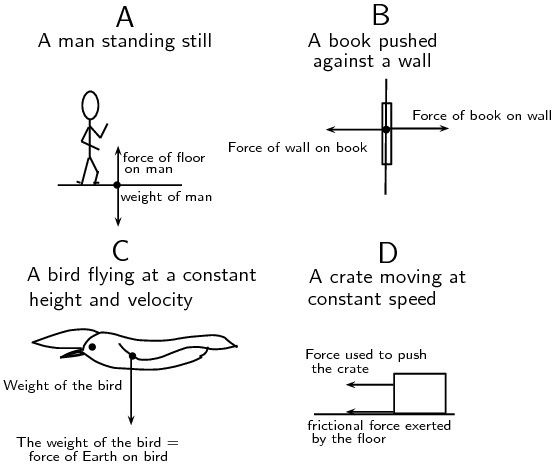A or B

### Forces in equilibrium (ESBKW)

At the beginning of this chapter it was mentioned that resultant forces cause objects to accelerate in a straight line. If an object is stationary or moving at constant velocity then either,

• no forces are acting on the object, or

• the forces acting on that object are exactly balanced.

In other words, for stationary objects or objects moving with constant velocity, the resultant force acting on the object is zero.

Equilibrium

An object in equilibrium has both the sum of the forces acting on it equal to zero.

temp text